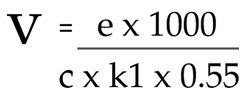# How many views do you need to make a living off YouTube?

Here's an effective method to calculate the number of views required to earn a certain amount of money on YouTube

The success of a YouTube channel is fueled by the ingenuity of the video content and is only limited by the imagination of its creator. One is tempted to start a YouTube channel after seeing the success of the average Joe, and there are misconceptions about how much much money there is to be made.

Though there is a correlation between the number of views and earnings, not all views are monetized and you only make money when an ad appears alongside the view. Revenue for these monetized impressions is calculated based on the CPM (cost per thousand ad impressions).

I have a simple mathematical formula to help you determine the number of views you need to earn a certain amount of money per month.Where ...

• V is the number of views, the output of the formula.
• e is your earnings. So for e.g. if you are expecting to earn \$2000, then that's the value of e
• c is the average CPM from ad impressions for your videos. This varies on several factors like the country of your viewers, age group, type of video etc. For e.g. if a bulk of your viewers are from India, you can expect an average of about \$2 CPM
• k1 is the % of views that are monetized. For e.g. if 30% of the views are monetized for ads, then k1=0.3
• 0.55 (a constant) in the formula is your share of the revenue, since YouTube retains 45% of the total ad revenue.

Let's apply the formula to a couple of scenarios ...

• Scenario 1: If you plan on making on making \$3000 per month from YouTube (assuming that is how much you need to make a comfortable living), here is how you would apply the formula ...

Here, e = \$3000, c = \$6 (this is usually the average CPM if your viewers are from around the world) and k1 = 0.4 (assuming that 40% of your views are monetized)

Applying this to the formula, the answer is approximately 2.3 million views per month or around 75,000 views per day.

• Scenario 2: Similarly, if wish to earn \$1000 per month from YouTube, and your target audience is predominantly Indian, here is how you would apply the formula ...

Here, e = \$1000, c = \$2 (this is usually the average CPM when your viewers are from India) and k1 = 0.3 (assuming that 30% of your views are monetized)

Applying this to the formula, the answer is approximately 3 million views per month or around 100,000 views per day.

I have applied the formula across a variety of cases and the results are fairly accurate (margin of error of around 10%) and you can apply your specific scenario to the formula to arrive at the number of views required.